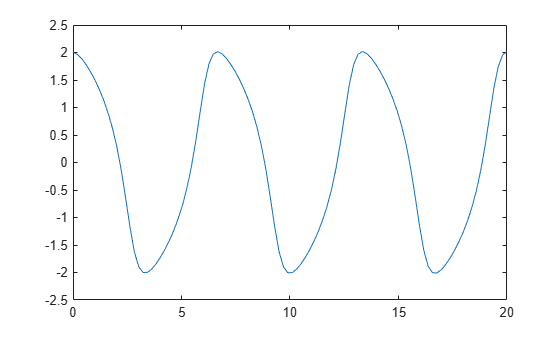# odeToVectorField

Reduce order of differential equations to first-order

Support for character vector or string inputs will be removed in a future release. Instead, use `syms` to declare variables, and replace inputs such as `odeToVectorField('D2y = x')` with ```syms y(x), odeToVectorField(diff(y,x,2) == x)```.

## Syntax

``V = odeToVectorField(eqn1,...,eqnN)``
``````[V,S] = odeToVectorField(eqn1,...,eqnN)``````

## Description

example

````V = odeToVectorField(eqn1,...,eqnN)` converts higher-order differential equations `eqn1,...,eqnN` to a system of first-order differential equations, returned as a symbolic vector.```

example

``````[V,S] = odeToVectorField(eqn1,...,eqnN)``` converts `eqn1,...,eqnN` and returns two symbolic vectors. The first vector `V` is the same as the output of the previous syntax. The second vector `S` shows the substitutions made to obtain `V`.```

## Examples

collapse all

Define a second-order differential equation:

`$\frac{{\mathit{d}}^{2}\mathit{y}}{{\mathit{dt}}^{2}}+{\mathit{y}}^{2}\mathit{t}=3\mathit{t}.$`

Convert the second-order differential equation to a system of first-order differential equations.

```syms y(t) eqn = diff(y,2) + y^2*t == 3*t; V = odeToVectorField(eqn)```
```V =  $\left(\begin{array}{c}{Y}_{2}\\ 3 t-t {{Y}_{1}}^{2}\end{array}\right)$```

The elements of V represent the system of first-order differential equations, where `V[i]` = ${{Y}_{i}}^{\prime }$ and ${Y}_{1}=y$. Here, the output `V` represents these equations:

`$\frac{\mathit{d}{\mathit{Y}}_{1}}{\mathit{dt}}={\mathit{Y}}_{2}$`

`$\frac{{\mathit{dY}}_{2}}{\mathit{dt}}=3\mathit{t}-\mathit{t}{\mathit{Y}}_{1}^{2}.$`

For details on the relation between the input and output, see Algorithms.

When reducing the order of differential equations, return the substitutions that `odeToVectorField` makes by specifying a second output argument.

```syms f(t) g(t) eqn1 = diff(g) == g-f; eqn2 = diff(f,2) == g+f; eqns = [eqn1 eqn2]; [V,S] = odeToVectorField(eqns)```
```V =  $\left(\begin{array}{c}{Y}_{2}\\ {Y}_{1}+{Y}_{3}\\ {Y}_{3}-{Y}_{1}\end{array}\right)$```
```S =  $\left(\begin{array}{c}f\\ \mathrm{Df}\\ g\end{array}\right)$```

The elements of `V` represent the system of first-order differential equations, where `V[i]` = ${{Y}_{i}}^{\prime }$. The output S shows the substitutions being made, S = ${Y}_{1}=f$, S = ${Y}_{2}$ = `diff(f)`, and S = ${Y}_{3}=g$.

Solve a higher-order differential equation numerically by reducing the order of the equation, generating a MATLAB® function handle, and then finding the numerical solution using the `ode45` function.

Convert the following second-order differential equation to a system of first-order differential equations by using `odeToVectorField`.

`$\frac{{\mathit{d}}^{2}\mathit{y}}{\mathit{d}{\mathit{t}}^{2}}=\left(1-{\mathit{y}}^{2}\right)\frac{\mathit{dy}}{\mathit{dt}}-\mathit{y}.$`

```syms y(t) eqn = diff(y,2) == (1-y^2)*diff(y)-y; V = odeToVectorField(eqn)```
```V =  $\left(\begin{array}{c}{Y}_{2}\\ -\left({{Y}_{1}}^{2}-1\right) {Y}_{2}-{Y}_{1}\end{array}\right)$```

Generate a MATLAB function handle from `V` by using `matlabFunction`.

`M = matlabFunction(V,'vars',{'t','Y'})`
```M = function_handle with value: @(t,Y)[Y(2);-(Y(1).^2-1.0).*Y(2)-Y(1)] ```

Specify the solution interval to be `[0 20]` and the initial conditions to be ${y}^{\prime }\left(0\right)=2$ and ${y}^{\prime \prime }\left(0\right)=0$. Solve the system of first-order differential equations by using `ode45`.

```interval = [0 20]; yInit = [2 0]; ySol = ode45(M,interval,yInit);```

Next, plot the solution $y\left(t\right)$ within the interval $t$ = `[0 20]`. Generate the values of `t` by using `linspace`. Evaluate the solution for $y\left(t\right)$, which is the first index in `ySol`, by calling the `deval` function with an index of 1. Plot the solution using `plot`.

```tValues = linspace(0,20,100); yValues = deval(ySol,tValues,1); plot(tValues,yValues)```Convert the second-order differential equation ${y}^{\prime \prime }\left(x\right)=x$ with the initial condition $y\left(0\right)=a$ to a first-order system.

```syms y(x) a eqn = diff(y,x,2) == x; cond = y(0) == a; V = odeToVectorField(eqn,cond)```
```V =  $\left(\begin{array}{c}{Y}_{2}\\ x\end{array}\right)$```

## Input Arguments

collapse all

Higher-order differential equations, specified as a symbolic differential equation or an array of symbolic differential equations. Use the `==` operator to create an equation. Use the `diff` function to indicate differentiation. For example, represent d2y(t)/dt2 = t y(t) by entering the following command.

```syms y(t) eqn = diff(y,2) == t*y;```

## Output Arguments

collapse all

First-order differential equations, returned as a symbolic expression or a vector of symbolic expressions. Each element of this vector is the right side of the first-order differential equation Y[i]′ = V[i].

Substitutions in first-order equations, returned as a vector of symbolic expressions. The elements of the vector represent the substitutions, such that `S(1) = Y`, ```S(2) = Y```,….

## Tips

• To solve the resulting system of first-order differential equations, generate a MATLAB® function handle using `matlabFunction` with `V` as an input. Then, use the generated MATLAB function handle as an input for the MATLAB numerical solver `ode23` or `ode45`.

• `odeToVectorField` can convert only quasi-linear differential equations. That is, the highest-order derivatives must appear linearly. For example, `odeToVectorField` can convert y*y″(t) = –t2 because it can be rewritten as y″(t) = –t2/y. However, it cannot convert y″(t)2 = –t2 or sin(y″(t)) = –t2.

## Algorithms

To convert an nth-order differential equation

`${a}_{n}\left(t\right){y}^{\left(n\right)}+{a}_{n-1}\left(t\right){y}^{\left(n-1\right)}+\dots +{a}_{1}\left(t\right){y}^{\prime }+{a}_{0}\left(t\right)y+r\left(t\right)=0$`

into a system of first-order differential equations, `odetovectorfield` makes these substitutions.

`$\begin{array}{l}{Y}_{1}=y\\ {Y}_{2}={y}^{\prime }\\ {Y}_{3}={y}^{″}\\ \dots \\ {Y}_{n-1}={y}^{\left(n-2\right)}\\ {Y}_{n}={y}^{\left(n-1\right)}\end{array}$`

Using the new variables, it rewrites the equation as a system of n first-order differential equations:

`$\begin{array}{l}{Y}_{1}{}^{\prime }={y}^{\prime }={Y}_{2}\\ {Y}_{2}{}^{\prime }={y}^{″}={Y}_{3}\\ \dots \\ {Y}_{n-1}{}^{\prime }={y}^{\left(n-1\right)}={Y}_{n}\\ {Y}_{n}{}^{\prime }=-\frac{{a}_{n-1}\left(t\right)}{{a}_{n}\left(t\right)}{Y}_{n}-\frac{{a}_{n-2}\left(t\right)}{{a}_{n}\left(t\right)}{Y}_{n-1}-...-\frac{{a}_{1}\left(t\right)}{{a}_{n}\left(t\right)}{Y}_{2}-\frac{{a}_{0}\left(t\right)}{{a}_{n}\left(t\right)}{Y}_{1}+\frac{r\left(t\right)}{{a}_{n}\left(t\right)}\end{array}$`

`odeToVectorField` returns the right sides of these equations as the elements of vector `V` and the substitutions made as the second output `S`.

## Version History

Introduced in R2012a

expand all